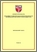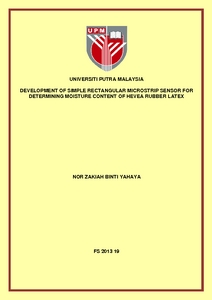# Development of simple rectangular microstrip sensor for determining moisture content of hevea rubber latex

## Citation

Yahaya, Nor Zakiah (2013) Development of simple rectangular microstrip sensor for determining moisture content of hevea rubber latex. PhD thesis, Universiti Putra Malaysia.

## Abstract

Various techniques have been proposed to determine the dry rubber content or moisture content in Hevea Rubber Latex (Hevea Brasiliensis). Unfortunately the techniques are either laborious, inaccurate, expensive or bulky. This thesis describes the design, fabrication, testing, analysis and validation of a microstrip patch sensor frequency range between 1 GHz and 4 GHz for determination of moisture content of Hevea Rubber Latex. Three different computational methods were used in the theoretical design and analysis of the microstrip sensor. The variational technique, Method of Moment and Finite Element Method were used to determine the resonance frequency and other parameters of the unloaded and latex loaded microstrip sensor. The measurement setup for determination of reflectivity consists of the microstrip patch sensor and an N5230A Agilent Professional Network Analyzer in the frequency range between 1 GHz and 4 GHz. All calibrations were performed using Agilent N4691-60004 (300 kHz - 26.5 GHz) Electronic calibration Module. Permittivity values for the Hevea Rubber Latex samples of different percentages of moisture content were obtained using both dielectric mixture model and a 4 mm open-ended coaxial line probe (Agilent 85070E). These permittivities were used as inputs to both variational and numerical methods to calculate the reflection coefficient of the unloaded and loaded sensor. The latter employed both Finite Element Method (FEM) and Method of Moment (MoM) implemented in 3D and 2-5D using COMSOL Multiphysics version 3.5 and Microwave Office version 7.03 software, respectively. FEM was utilized to investigate electric fields distribution in the samples. Comparison of results between the measured and calculated resonance frequency, magnitude and phase of the reflection coefficient, phase shift, frequency shift, conductance and susceptance for a range of latex samples of different percentages of m.c from 36 % to 86 % have been discussed in this work. It was found that the FEM provided the most accurate results. Calibration equations have been established to predict the amount of moisture content from the measured microwave parameters. The equations were validated by comparing the results with the actual moisture content obtained using the standard oven drying method. The calibration equations based on the magnitude and conductance measurements were found to be most accurate at 3 GHz and 3.2 GHz, respectively whilst both the phase shift and susceptance were at 4 GHz. However, the calibration equation with lowest mean percentage error 2.0 % was found when using the frequency shift of the peak of the magnitude of the reflection coefficient.Preview
PDF
FS 2013 19 IR.pdfView Item